## MetaQuotes

6 October 2023

Successful trading in financial markets requires thoughtful and well-founded decision-making. Comprehensive performance analytics can assist traders in making informed investment decisions and in optimizing their strategies. In this article, we will look at five key performance indices which characterize trading efficiency and stability.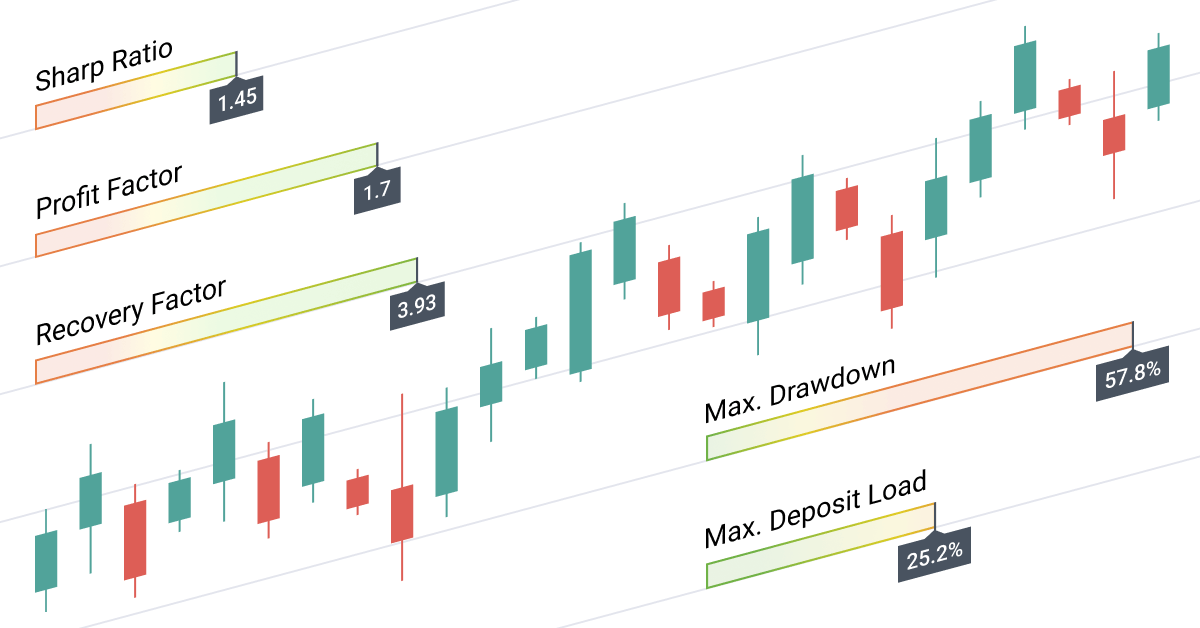### 1. Sharpe Ratio

• What it is: A measure of strategy profitability in relation to risk.
• Why it is important: A high Sharpe Ratio value indicates that a strategy can efficiently generate profits with minimal risks. The higher the ratio, the better.
• How to interpret: A value greater than 1 indicates that the strategy's returns justify the associated risks. Ratio values of 3 or higher indicate an excellent strategy.

Below is a successful trading report which shows an annual growth of more than 1,500%. Balance and growth diagrams are quite smooth, without sharp drops and spikes. However, the strategy cannot be considered stable since the Sharpe Ratio is 0.21, which means that the trader is taking very high risks to achieve these trading results.

The Sharpe ratio is interpreted as follows:

• Sharpe Ratio < 0                Poor. The strategy is generally unprofitable.
• Sharpe Ratio  < 1.0            Undefined. The risks are not justified. The use of such strategies can only be considered when there are no alternatives.
• Sharpe Ratio ≥ 1.0             Good. If the Sharpe Ratio is higher than one, it means that the risks are justified by the portfolio/strategy performance.
• Sharpe Ratio ≥ 3.0             Excellent. A high ratio indicates lower probability of losses in each individual trade.

If the Sharpe ratio is less than zero, the strategy is unprofitable. However, even without analyzing the Sharpe Ratio, such poor results can be evident from balance and growth diagrams only.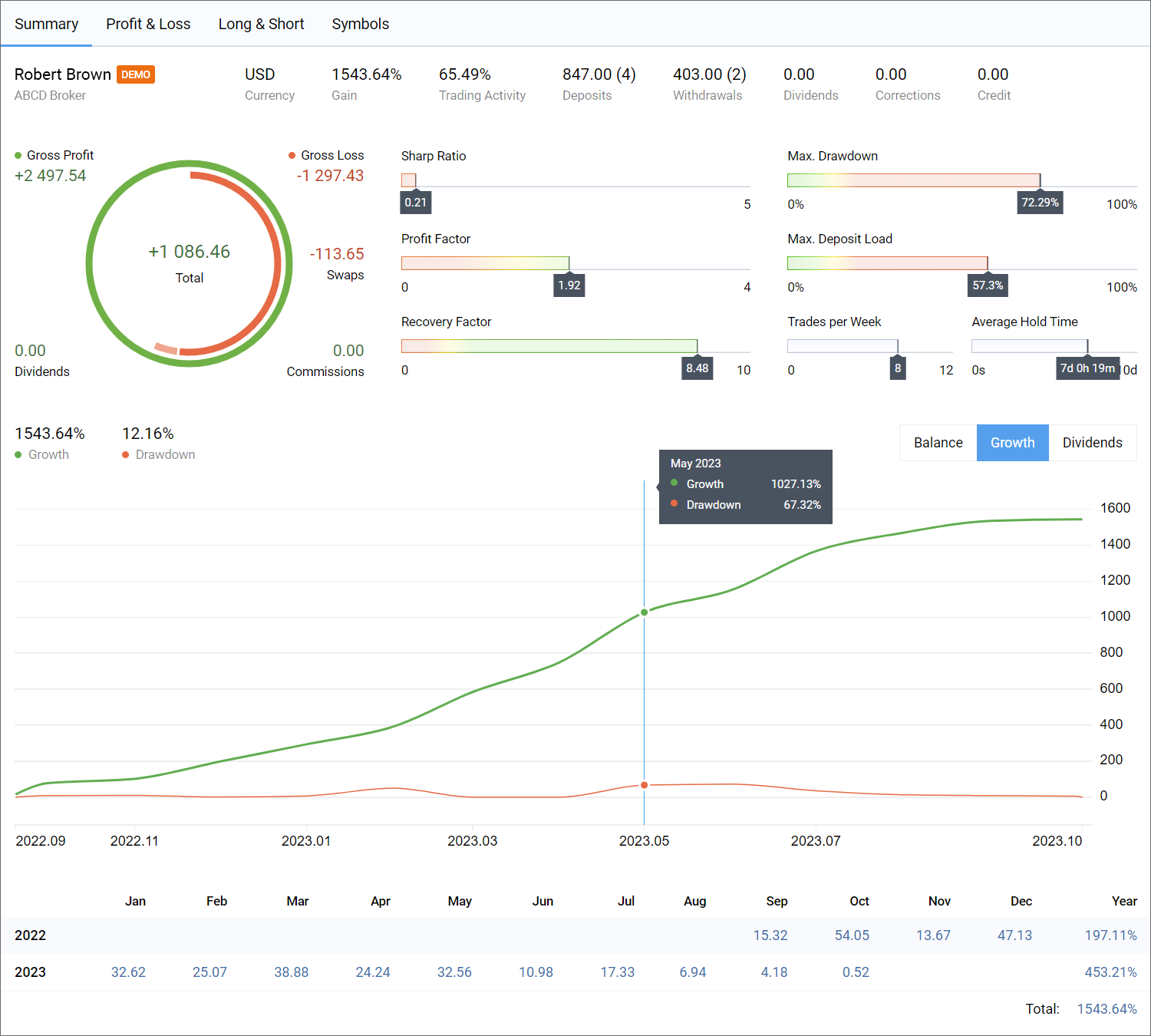### 2. Maximum Drawdown

• What it is: The maximum decline in balance from its highest value.
• Why it is important: The metric shows the portion of capital at risk during the worst trading period.
• How to interpret: Lower drawdown values indicate a more reliable strategy.

The maximum drawdown in the above report is equal to 72.3%, which indicates a very high risk. The diagram above shows a drawdown of 67% in May 2023. This risk value is considered extreme since the optimal drawdown value should not exceed 20-30%.

Why should the optimal drawdown be no more than 30% (preferably less than 20%)? When choosing a trading strategy, you should assume that the historically registered drawdown can actually be surpassed by a factor of 2 or more. If the maximum drawdown is 30%, the future value can reach 60% (2*30%). A trading account can endure such a drawdown value. However, if the maximum drawdown is 72%, then it may well be 100% in the future, which means that all the funds could be lost.

### 3. Recovery Factor

• What it is: The ratio of generated profit to maximum drawdown.
• Why it is important: The factor shows the ability of the strategy to recover from losses.
• How to interpret: The higher the Recovery Factor, the faster the strategy covers losses and begins to generate profits.

The Recovery Factor in the above report is equal to 8.26 and is in the green zone, which is a good value. Ideally, a trading strategy should have a Recovery Factor greater than 3, with greater values being more desirable.

The Summary report in the funds mode shows that the account equity (USD 333.83) is significantly lower than the balance (USD 558.29). The account had a large drawdown, but the resulting profit provided a good recovery factor.

A strategy with a high Recovery Factor (3 or more) indicates that the current drawdown can be compensated by future profits. If the Recovery Factor is less than 1, then several consecutive drawdowns may potentially cause the account balance to drop to zero.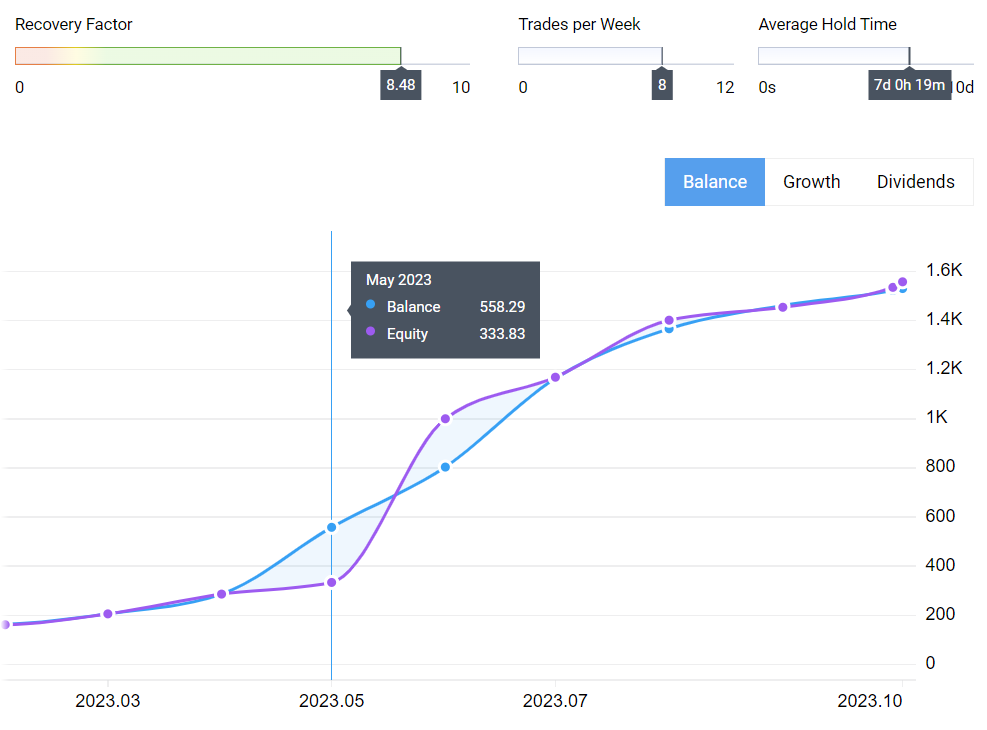### 4. Profit Factor

• What it is: The ratio of total profits to total losses.
• Why it is important: The factor shows the potential long-term efficiency of the strategy.
• How to interpret: Values greater than 1 indicate profitability.

The primary purpose of financial trading is to generate profits. While any trading strategy may have winning and losing trades, profits from winning trades should exceed losses from unprofitable ones. The Profit Factor precisely indicates how much the total generated profits exceed the total losses.

The Profit Factor in this report is equal to 1.91, which is a good value. Accordingly, the diagram is in the green zone.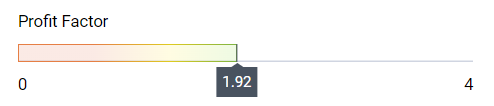• What it is: The highest percentage of account funds utilized for open positions.
• Why it is important: High deposit loads carry additional risks for a trading account. If the market moves in an unfavorable direction, the probability of losing all funds increases if the deposit loads are high.
• How to interpret: Lower values indicate reduced exposure to potential losses.

There is no optimal deposit load value which is suitable for all strategies. The load depends on the specific trading style and position holding times. Scalping strategies can utilize higher deposit shares since they aim at generating profits from small price changes and usually open increased-volume positions.

For margin trading, including Forex, the margin requirement is significantly lower due to the provided leverage. This allows for increased profits from small price changes but at the same time carries higher risks of potential losses if the currency pair moves in an unfavorable direction. For example, if the leverage is 100, then the margin required to open a position is reduced by 100 times. Consequently, if a trader opens a position for the entire deposit and the price moves 100 points in the opposite direction, the entire deposit will be lost. Furthermore, many brokers set a Stop Out level of 50%, which means that 50 points in an unfavorable direction will lead to large losses. Such a movement is typically within the daily range for most currency pairs.

The maximum deposit load in this report is 57%. Such trading is risky and thus the metric is in the red zone.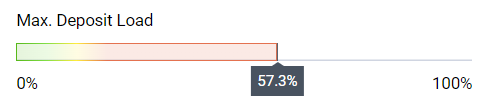### Summary

The Summary page features key indices, including balance/equity graphs and growth percentage dynamics. Analysis is summarized as below:

• Growth and balance graphs are quite smooth, without sharp fluctuations — ✔️
• Sharpe Ratio = 0.21 — X
• Maximum Drawdown = 72% — X
• Recovery Factor = 8.26 — ✔️
• Profit Factor = 1.91 — ✔️
• Max. Deposit Load = 57% — ✔️

While three out of the five metrics are promising, the trading strategy cannot be considered stable and safe. The generated profits are associated with high risks.

All of the considered statistics are important for the analysis and evaluation of trading strategies. You should always analyze a combination of performance indices, taking into account the specific trading strategy features, and market conditions. Proper use and understanding of statistical metrics can assist in minimizing risks and in improving trading results.

With the updated MetaTrader 5 trading report, traders can evaluate their strategy performance directly in the terminal. Such comprehensive analytics can highlight strategies' positive aspects, and prompt at weak areas, which if improved can reduce risks and enhance trading stability.